# Combined Gas Law Calculator

Created by Wojciech Sas, PhD candidate
Reviewed by Bogna Szyk
Last updated: Dec 07, 2021

Combined gas law calculator is a great tool to deal with problems related to the most common transformations of gases. Read about isobaric, isochoric, isothermal, and adiabatic processes of ideal gases (gases that can be described by the ideal gas equation). And how it is possible for the ideal gases to do work or release/absorb heat. Check out the exact values for real gases and forget about struggling with thermodynamic exercises!

## What are thermodynamic processes? Combined gas law formula

An ideal gas can be described by several parameters, which are pressure p, volume V, temperature T and the amount of particles n. They are correlated with the equation: p * V = n * R * T, where R stands for ideal gas constant and equals 8.3144598 J/(mol * K).

During any process at least two of these properties change which can be compiled in combined gas law formula: p * V / T = k, where k is a constant.

Out of all transformations, we can distinguish a few which encompass a vast majority of examples from everyday life, or they can be treated as good approximations. In this combined gas law calculator, we consider processes in which the number of particles is constant, thus we can imagine a gas in a closed container. These are:

• isochoric process,
• isobaric process,
• isothermal process,

## First law of thermodynamics

Internal energy U is the sum of all kind of energies that are present in a system. It's quite tricky to estimate the precise value of internal energy, but it is possible to find thermal energy changes ΔU, which are described by the first law of thermodynamics: ΔU = Q - W, where Q denotes heat absorbed, and W is work done by gas. Internal energy change is proportional to temperature variation ΔT and type of gas with the following equation: ΔU = Cv * n * ΔT, where Cv is molar heat capacity under constant volume. For ideal gas it takes values:

• 3/2 * R for monoatomic gas,
• 5/2 * R for diatomic gas,
• 3 * R for gases with more complex molecules.

In real gases, these parameters differ from theoretical ones, but it's already contained in our thermodynamic processes calculator. The general formula for work done by the gas is expressed as: ∫p(V)dV if we consider pressure as the function of volume. Although it isn't trivial in general, you can check how the formula simplifies for processes mentioned below.

## Isochoric process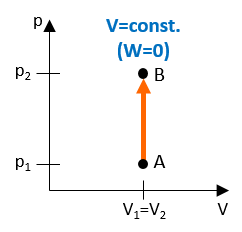During this transition volume is a constant parameter, so that initial properties p₁, T₁ changes to p₂, T₂ as follows: p₁ / T₁ = p₂ / T₂. The invariability of volume means that gas doesn't do any work and the heat absorbed by gas is precisely the same as internal energy change: ΔU = Q = Cv * n * ΔT. This process can be visualized for gas kept in a rigid container, but which can exchange heat with an environment. Gay-Lussac's law corresponds to this thermodynamic process.

## Isobaric process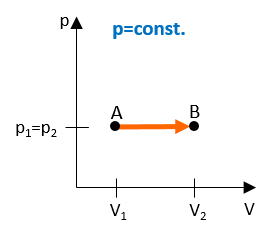It is assumed that pressure is a constant gas parameter during this transition. Therefore the initial parameters V₁, T₁ transform to V₂, T₂with the following form of combined gas law formula: V₁ / T₁ = V₂ / T₂. Due to the fact that pressure is invariant, the formula for work done by the gas is given by: W = p * ΔV. Heat, however, can be calculated as: Q = ΔU + W = Cv * n * ΔT + p * ΔV = Cp * n * ΔT. Cp is known as molar heat capacity under constant pressure, and for an ideal gas is associated with Cv, so that Cp = Cv + R. Charles' law is related to this transition.

## Isothermal process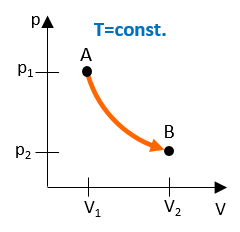The constant parameter in this transition is temperature so that initial properties p₁, V₁ change to p₂, V₂, and the correlation is: p₁ * V₁ = p₂ * V₂. In the presented example we can see that, according to the ideal gas equation, the pressure is the following function of volume: p(V) = n * R * T / V = A / V, where A is constant throughout the whole process. The general expression for work done by the gas can be estimated as: W = n * R * T * ln(V₂ / V₁), where ln states for natural logarithm of a given number. Due to the constancy of temperature, initial energy doesn't change, which implies the equality: Q = W. One can say that the entire heat absorbed by gas is transformed to work done by it, but on the other hand, this transition is known to be very slow in practice. Boyle's law describes thermodynamic processes of this kind.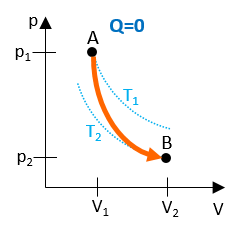In this transition all three parameters change, but simultaneously the gas doesn't exchange heat with the environment. The following formula is valid: p₁ * V₁γ = p₂ * V₂γ, where γ = Cp / Cv is known as heat capacity ratio. The work done by the gas is opposite to its initial internal energy change W = -ΔU. This process describes transitions which progress rapidly, and there is no time for the gas to absorb or release any heat.

## Computational example

Let's assume that you want to find the internal energy change, the heat absorbed and the work done by nitrogen stored inside a flexible container of volume 0.5 m³ under atmospheric pressure and at temperature 250 K, which is heated up to 300 K. In this case, we consider an isobaric process.

• Calculate the final volume: V₂ = V₁ * T₂ / T₁ = 0.5 m³ * 300 K / 250 K = 0.6 m³,

• Work out the number of molecules: n = p * V₁ / (R * T₁) = 101.325 kPa * 0.5 m³ / (8.314 J/(mol * K) * 250 K) = 24.375 mol,

• Find the heat capacity Cv of nitrogen, which is 20.814 J/(mol * K) (for ideal diatomic gas it should be equal to 20.786 J/(mol * K)),

• Estimate internal energy change: ΔU = 20.814 J/(mol * K) * 24.375 mol * 50 K = 25.367 kJ,

• Determine the work done by gas: W = 101.325 kPa * 0.1 m³ = 10.133 kJ,

• And evaluate the heat absorbed by nitrogen: Q = 25.367 kJ + 10.133 kJ = 35.500 kJ

You can always save your time and use our combined gas law calculator!

## Carnot cycle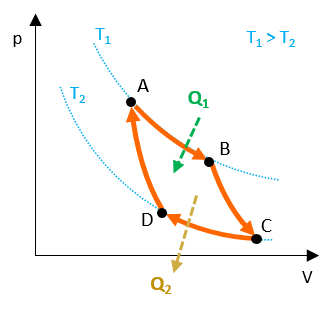This is one of the cycles which represents the model of an ideal engine with the highest efficiency possible in the mean of thermodynamic laws. It consists of two adiabatic and two isothermal processes. This engine absorbs heat from hot reservoir, transforms it into work and release the rest of heat to the cold one. With this combined gas law calculator, you can design any kind of thermodynamic cycle and find out how this change influences the output efficiency!

Wojciech Sas, PhD candidate
Select process and gas type
Process
Working gas
Nitrogen
Insert initial parameters
Initial pressure (p₁)
Pa
Initial volume (V₁)
Initial temperature (T₁)
K
Resulting parameters
Final pressure (p₂)
Pa
Final volume (V₂)
Final temperature (T₂)
K
Internal energy change (ΔU)
J
Work (W)
J
Heat (Q)
J
People also viewed…

### Car vs. Bike

Everyone knows that biking is awesome, but only this Car vs. Bike Calculator turns biking hours into trees! 🌳

### Photon detection efficiency (SiPM)

Photon detection efficiency calculator is a handy tool that allows for a quick conversion between responsivity and Photon Detection Efficiency (PDE) in Silicon Photomultipliers (SiPMs).

### Snowman

The perfect snowman calculator uses math & science rules to help you design the snowman of your dreams!

### Thermal expansion

The thermal expansion calculator finds the change in length or volume of a material subject to extreme temperatures.